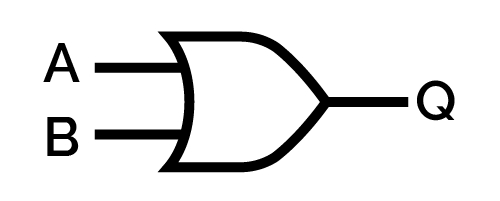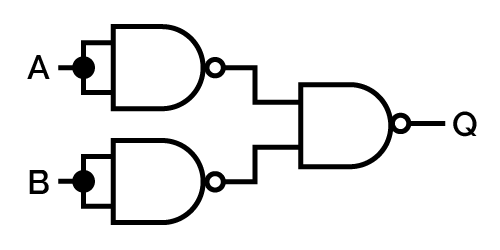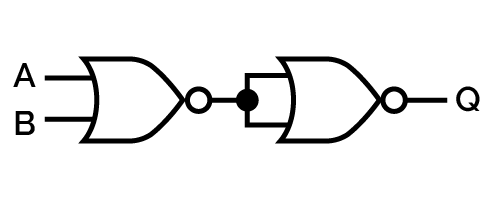# OR Calculator

Created by Davide Borchia
Reviewed by Anna Szczepanek, PhD and Steven Wooding
Last updated: Jun 01, 2023

• What is the logical OR operation?
• How to calculate the OR in the binary numeral base.
• How to use our bitwise OR calculator.

Plus, much more!

## What is the logical OR? — disjunction in logic and grammar

As for the English language, the logical OR operation connects two or more statements (in grammar and programming), quantities (in mathematics and logics), in general, the operands with a disjunction. The outcome of the operation is true in all possible combinations of the veracity of the statements unless all the operands are false. In this case, the result is false too.

With excessive specificity, we can also call the logical OR inclusive logical OR, as it results in a true statement when both (all) operands are true. The operation where the disjunction of two true statements results in a false statement is similarly called exclusive logical OR.

🙋 You can meet the exclusive logical OR at the xor calculator!

We represent the logical OR in many different ways:

• In logic the symbol $\lor$, as in $A\lor B$.
• In programming the symbols $||$ is usually preferred in conditional statements.
• In electronics, the symbol $+$ reminds us of the relationship between logic and binary operations.

The operands of the logical OR can be any set of quantities with two possible values. For example:

• Boolean variables, with possible values true and false; and
• Binary digits that can assume values $0$ and $1$.

The logical OR has multiple properties as all mathematical operators:

• It is associative: when calculating the OR logical operation on more than two operands, the order with which we group them doesn't matter.

• It is commutative, which means that the order of the operands doesn't matter.

• It is distributive: you can apply it to the operands of other binary operations or their results without changing the result.

• It preserves the overall integrity of the operands: if both operands are true, the result is true; if both operands are false, the result is false (truth/falsehood preservation).

We can see the operation of the logical OR by looking at the truth table:

$\text{\textbf{in}}_\bold{1}$

$\text{\textbf{in}}_\bold{2}$

$\text{\textbf{output}}$

false

false

false

false

true

true

true

false

true

true

true

true

## The OR logical gate

The operations of the logical OR translate into practice in logical circuits thanks to the logical OR gate. This type of device has wide use in electronics and circuitry. The symbol of the OR logical gate is:Where the two inputs represent the two operands, we need to use a set of other logic gates to build the OR gate, depending on which one we choose as the fundamental gate.

If we choose the NAND logic, the OR gate would result from the appropriate connection of three NAND gates.In the NOR logic, we simply need a couple of NOR gates, which are nothing but the negation of the OR gate.## How to calculate the OR in the binary numeral system

To calculate the OR logical gate in the binary number system, we assign to the operands the values $0$, and in the alternative, $1$. Applying the logical OR to two binary digits roughly corresponds to summing the two digits. However, if we perform $1+1$, since we would get $10$ as a result, we sum again, thus obtaining $1+0=1$ as the final result: the sum of two ones becomes a one itself.

When dealing with numbers with multiple digits, we calculate the bitwise OR, which is nothing but applying the OR logic to all the possible digits.

To do so, calculate the OR, bit by bit, starting from the right side of the numbers.

## Example calculation of the OR logical gate

Take two numbers in the binary numeral base, and calculate the OR operation between them. Let's say that you choose $101011$ and $11010$. Follow these steps:

1. Align the numbers on the right:
$\quad\quad \begin{split} 101011&\\ 11010& \end{split}$
1. Start computing the OR operation on the rightmost bit: $1\lor0=1$.

2. Move to the left, and calculate the bitwise OR for all digits:

• $1\lor1=1$;

• $0\lor0=0$;

• $1\lor1=1$; and

• $0\lor1=1$.

3. If there are more digits in an operand, copy them as they are.

4. Write down the results in the proper order: $111011$.

Don't worry if the number of $1$s in your results is higher than in the original operands: as three out of four combinations of digits have this result, it's expected!

## How to use our bitwise OR calculator

Our OR calculator implements this logic operation for binary numbers. We included both negative and positive numbers using the one's complement method (you can meet it at our one's complement calculator). The choice of the binary number representation restricts the possible numbers you can input: if you desire larger inputs, increase the bits in the word in the first variable. With the second variable, you can choose the numeral system in which you want to input your operands: binary, octal, and decimal!

After you input your number, we will show you the binary conversions of the operands and the results of the logical OR in all numeral systems you can choose above.

🙋 Visit our bitwise calculator for a compendium on logical operations. Check out the more specific AND calculator for a detailed view of one of them!

## FAQ

### What is the logical OR operator?

The logical OR operator is a logical operation between two quantities corresponding to an inclusive disjunction: the result of the OR operation is true in all possible combinations of inputs apart from the one where both quantities are false. You can compare the logical OR to the binary addition. However, as we can have only a digit as a result, if we are summing 1 and 1, we consider the result to be 1 instead of 10.

### How do I calculate the logical OR operator?

To calculate the logical OR between two binary numbers:

1. Align the numbers to the right.
2. Start calculating the bitwise OR of every pair of digits, and note the result in the proper position.
3. If one number is longer than the other, copy the excess digits as they are.

You are done!

### What is 10110 logic OR 11101?

The result of the logic OR operation 10110 ∨ 11101 is 11111. To find this result, follow these easy steps:

1. Align the two numbers to the right.
2. Start considering digits in the same position: sum the bits and write down the result. If the result is 10, take 1 as the result.
3. The first OR operation would be 0 + 1 = 1, the second one 1 + 0 = 1, etc.
4. Once you reach the last digit, you are done!

### How do I calculate OR with three inputs?

To calculate the OR logic operation with three inputs, we use the associative property of the operation. Let's consider the inputs A, B, and C. You can write the operations in two ways: (A ∨ B) ∨ C and A ∨ (B ∨ C). The two expressions are equivalent.

Perform the OR operation on the desired pair of inputs, then calculate the logical OR between the last input and the result.

Davide Borchia
Input data
Binary representation
8-bit
Datatype
Decimal
You can enter decimal numbers between -128 and 127.
Number 1
Number 2
People also viewed…

Addiction calculator tells you how much shorter your life would be if you were addicted to alcohol, cigarettes, cocaine, methamphetamine, methadone, or heroin.

### Korean age

If you're wondering what would your age be from a Korean perspective, use this Korean age calculator to find out.

### Long subtraction

This long subtraction calculator helps you solve any long subtraction problem for any two whole numbers as well as for numbers with decimal places.

### Orthocenter

With this orthocenter calculator you'll quickly find out the coordinates of this important triangle center.